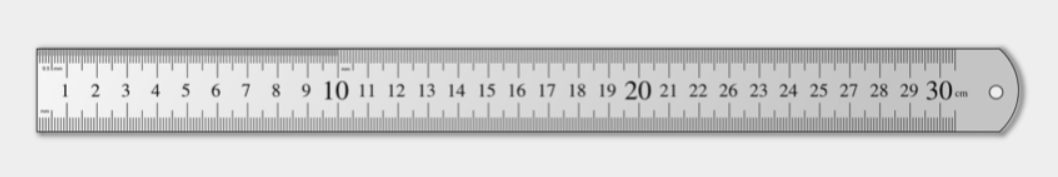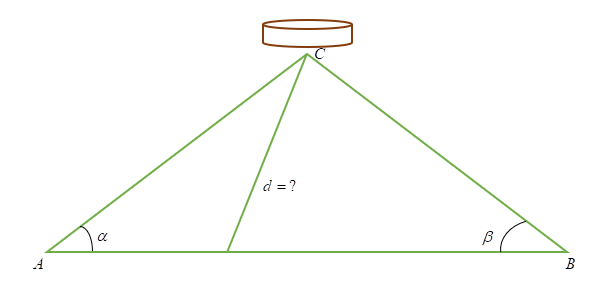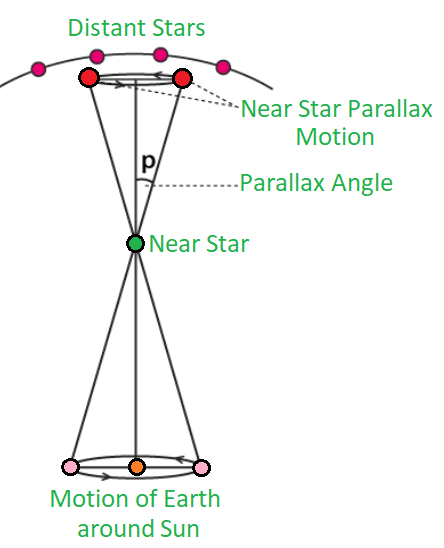Length Measurement

• Last Updated : 21 Jul, 2021

Previously, length was measured using units such as the length of a foot, the breadth of a palm, and so on. The ‘Cubit’ was one of the first means of measuring length. It is the length of the arm from the elbow to the tip of the fingers. These units vary from person to person, resulting in non-uniform measures.

How can we know how far the moon is from the earth or how far the moon is from the sun? How did we determine the earth’s diameter? Measuring length isn’t always simple or easy. We’ll try to respond to these queries in the sections below. In addition, we will learn about the many methods for measuring length.

Length

The measurement or amount of anything from one end to other is referred to as length.

In other terms, it is the largest of the two or the highest of three geometrical form or item dimensions. The width and length of a rectangle, for instance, are its dimensions. Furthermore, under the International System of Quantities, length is a quantity with the dimension distance.

The meter, abbreviated as m, is the basic unit for length in the International System of Units (SI). The length or distance is measured in kilometers (km), meters (m), decimeters (dm), centimeters (cm), and millimeters (mm) in the metric system (mm). It is possible to convert quantities from meters to centimeters, kilometers to meters, centimeters to millimeters, and so on.

Measurement of Length

There were no modes of transportation available in ancient times. People used to travel on foot or by using animals to transport goods. Over time, the term “wheel” was coined. This signified a significant shift in human forms of transportation. Since then, new forms of transportation have been invented and improved regularly. The steam engine was created, and it had a great influence and was instrumental in shaping the world as we know it today.

As a result, transportation has a lengthy history. Did the folks have any idea how far they had to go? To go to any location, one must first determine how far away it is. This aids in deciding whether to walk, take the train, bus, or fly to that location. To determine how far apart two locations are, we must first determine the distance between them. But what exactly does measurement imply? What is the best way to measure a physical quantity? The comparing of an unknown amount to a known amount is known as measurement. A numerical number known as “magnitude” and a “unit” are used to indicate the outcome of the measurement. A ‘unit’ is a pre-determined unit of comparison against which other physical quantities are measured.

The length of the foot, the breadth of the palm, and other such quantities were used to measure the length in the past. The ‘Cubit’ was one of the first means of measuring length. It is the length of an arm measured from elbow to tips of fingers. These units varied from person to person, resulting in non-uniform measures. A set of standard units of measurement has been recognised all around the world to preserve uniformity in measurements.

The International System of Units (SI) is one of the most widely used measurement systems in the world. The basic unit of length in the SI system is the meter. The C.G.S. system is another system of units in which the centimeter is the basic unit of length.

Conventional Methods of Measurements

Historically, the human body served as the foundation for length units.

• Inch: An inch is a unit of measurement that was once used to measure the length of little things such as the length of paper, the seam of cloth, and so on.
• Foot: A foot is a unit of length that is commonly defined as 15.3 per cent of a human body’s height, with an average height of 160 cm. This unit differed from one location to the next and from one transaction to the next. The Romans and Greeks favored this unit, which was commonly used to compute the height of humans and livestock, the size of a piece of fabric, the size of a structure, and so on.
• Cubit: A cubit is a unit of length based on the length of the forearm, which is commonly measured from the tip of the middle finger to the forearm’s length bottom of the elbow. The Egyptians and Mesopotamians favored this unit. Cubit rods have been unearthed among the ancient Egyptian civilization’s relics. These rods are typically 20 inches long and are split into seven palms, each of which is split into four fingers, which are further subdivided.
• Yard: A yard is a measurement of distance based on human paces. It is usually measured in two cubits, which is around 36 inches.
• Mile: A mile is equal to 1,000 paces, where a pace is equal to two steps and the walker returns to the same foot.

A foot is 12 inches long, and a yard is three feet long. It was simple to describe how distant the next village was and to determine if an object would fit through a doorway using these dimensions. These dimensions also made it easier for individuals to swap garments and wood.Scale

Triangulation Method

Let’s take a closer look at what the triangulation approach entails. How might triangulation assist us in determining the distances between distant stars? The parallax approach makes use of the fact that a triangle may be entirely defined using only three parts. Triangulation is the process of determining the values of a triangle to determine an item’s position. Surveyors and architects frequently employ such techniques.

Triangulation is the method of identifying the location of a point by calculating the angles to it from two known sites rather than calculating distances directly.

Triangulation Example

Let’s put this into practice with a real-life scenario. How can we estimate a large object distance from any distance without actually measuring it? It may be measured using the triangulation method.Triangulation Method of measurement

• Let’s start by constructing a fixed baseline with two points AB.
• The angle formed by point A concerning the object is denoted by α, whereas the angle formed by point B concerning the object is marked by β.
• Now that we have the baseline AB and the angles, we can determine the remainder of the triangle’s attributes, such as the position of the third point, which is the object.

Parallax Method

The displacement or shift in the apparent location of an item when observed from two distinct points of view is known as parallax.

The two places of vision each have their own line of sight, and parallax is defined as half the angle between them. When you’re driving in a car and glance about, you’ll notice that items far away appear to move more slowly than items closer to you. This is the parallax effect. Because the parallax of nearby objects is greater than that of distant ones, the parallax may be utilised to measure distances.

When the phenomena of parallax is coupled with triangulation, the position of the item may be determined with great precision. The parallax method is commonly used by astronomers to determine the distances between stars.

Distance Measurement by Parallax Method

The principle of triangulation is used to the measuring of distance through parallax. We learned from triangulation that a triangle may be completely specified if two angles and sides are known.

The distance of a faraway star is being computed in the image below. The star closer to Earth than the farthest one gives the limited parallax value. By observing the star from two known places on Earth that form the triangle’s baseline, we may determine the value of the parallax angle.Parallax Method

Let’s denote the parallax half-angle between two places on Earth ‘p.’ The radius of the Earth is the greatest value of ‘d,’ and the distance of the star may be considered to be just slightly more than that of the sun. Because the distance from the sun is several orders of magnitude lower than the radius of the Earth, the parallax angle we obtain is exceedingly modest.

Application

The distance to an object measured in parsecs (in terms of light speed) is equal to the reciprocal of parallax angle measured in arcseconds.

Relation between the distance of a star, and its parallax is given as:

D = 1 ⁄ p

where D is the distance of star and p is the parallax angle.

To solve the difficulty of tiny ratios, the parallax of a star is most commonly estimated using yearly parallax, which is defined as the difference in a star’s location as seen from the Earth and the Sun. Instead of using the Earth’s radius as a fixed baseline, the radius of the Earth’s revolution around the Sun is used, which increases the size of the baseline and hence the top angle, making it simpler to measure.

However, for any celestial objects near to the Earth, we can consider the diameter of the Earth as a baseline, and the distance of any celestial objects is given as:

x = b ⁄ θ

where x is the distance of the object from the Earth, b is the baseline or diameter of the Earth and θ is the angle subtended by the object.

Sample Problems

Problem 1: If a person covers 1.5 yards in one step, how much distance will he cover in 30 steps?

Solution:

Given:

Total number of steps, n = 30

Value of 1 step, d = 1.5 yards

Total distance covered by the person, D = n d

= 30 × 1.5 yard

= 45 yards

Hence, the distance covered by the person is 45 yards.

Problem 2: Astronomers apply which method to determine how far away a star is?

Astronomers use parallax to calculate the distance between stars. Trigonometric parallax is another name for parallax.

Problem 3: What is parallax?

The two items appear to be coincident when seen in a straight line. There is a relative displacement between the things if they are at separate locations and the eye is shifted sideways. The closer item travels in the opposite direction from the eye, whereas the further object travels in the same direction.

When two things are perceived in a straight line and the eye is shifted to the side, this is referred to as parallax.

Problem 4: What was the conventional method of measuring the length?

The length was measured in history with the help of human body. These were based on the several methods like distance from tip of middle finger to bottom of elbow, or human paces or human heights, etc. However, it was discarded later because these methods were different for different countries and were limited to measure the long distances.

Problem 5: The Moon subtends an angle of 1° 55’ at the baseline equal to the diameter of the Earth. What is the distance of the Moon from the Earth? (Radius of the Earth is 6.4 × 106 m)

Solution:

Given:

The angle subtended by moon, θ = 1° 55’ = 115’

We know, 1’ = 60’’ and 1’’ = 4.85 × 10-6 rad

Therefore, 115’ = (115 × 60)’’ × 4.85 × 10-6 rad = 3.34 × 10-2 rad

The baseline for the Moon is the diameter of the Earth, b = 2 × 6.4 × 106 m = 1.28 × 107 m

Distance of the Moon from the Earth, x = b ⁄ θ

= 1.28 × 107 m ⁄ 3.34 × 10-2 rad

= 3.83 × 108 m

Hence, the distance of the Moon from the Earth is 3.83 × 108 m.

My Personal Notes arrow_drop_up